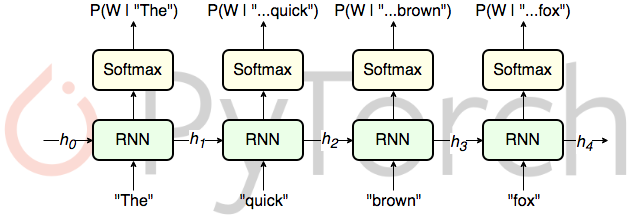Categories:

Updated:

# What is a Language Model?

Given a sequence of words $y^{<1>},…,y^{<T_y>}$ , a language model computes how likely this sequence would exist; $p(y^{<1>},…,y^{<T_y>})$ .

For example, a language model would say that p(‘pair of shoes’) is bigger than p(‘pear of shoes’).

How do we build Language Model with RNN?

# Collect training data

Our training set will be a large corpus(set) of english text.

# Tokenization

First, we tokenize words into vectors. Usually, we add <EOS>(end of sentence) and <UNK>(unknown) tokens to our vocabulary. So if there are $n$ words in our vocabulary, each of the word vector would have $n+2$ elements.

Also, we can decide on whether to get rid of punctuations.

For example, let’s say we want to tokenize sentence ‘I like Sarah.’ and don’t consider punctuations. Sarah isn’t in our vocabulary. Then our sequence will be $[y^{<I>}, y^{<like>}, y^{<UNK>}, y^{<EOS>}]$ , where each $y$ will be a vector representation of each word.

### Character Level Language Model

To get rid of ‘unknown words’, we might consider using ‘character level language model’. That is, we can construct our vocabulary with characters.(e.g. [a,…,Z,0,…,9])

However, this is computationally expensive, and since the model becomes long, front units are hardly recognized by the output.

# RNN ModelFirst, set $x^{<1>}$ and $a^{<0>}$ vectors composed of 0s. Then, we pass it to our RNN architecture.

$y^{<t>}$ , which is a softmax of $W_{ya}a^{<t>}+b_y$ , will be a probability distribution that a word will appear in $t$th sequence, given previous words. For example, $\hat{y}^{<2>}$ actually means $p(y^{<2>}|y^{<1>})$ (probability words will appear in 2nd place given 1st word).

We randomly pick a word from distribution of $y^{<t>}$ and the picked word will become $x^{<t+1>}$ to be feeded into the next sequential layer.

From this RNN model, we can know two things.

First, we can know probability an input sentence exist. We can multiply all of the output $\hat{y}$ s to compute that probability.

Second, we can know probability each word will come next given initial $t-1$ sequence of words. That is particularly $\hat{y}^{<t>}$ . For example, we can know that a word ‘school’ has higher probability than a word ‘snake’, when predicting what word will come next after ‘I went to’.

# Loss Function

where $k$ represents class and $t$ sequence number.

# Sampling a Sequence from RNN Language Model

From a trained RNN language model, we can sample a likely sentence.

First, randomly pick the first word from distribution $\hat{y^{<1>}}$. Then input the picked word vector as $x^{<2>}$ to the next sequential layer. Use $\hat{y^{<2>}}$ which was computed with $a^{<1>}$ and $x^{<2>}$ to sample second word. Repeat this until you come to sample <EOS> from distribution.

Categories: